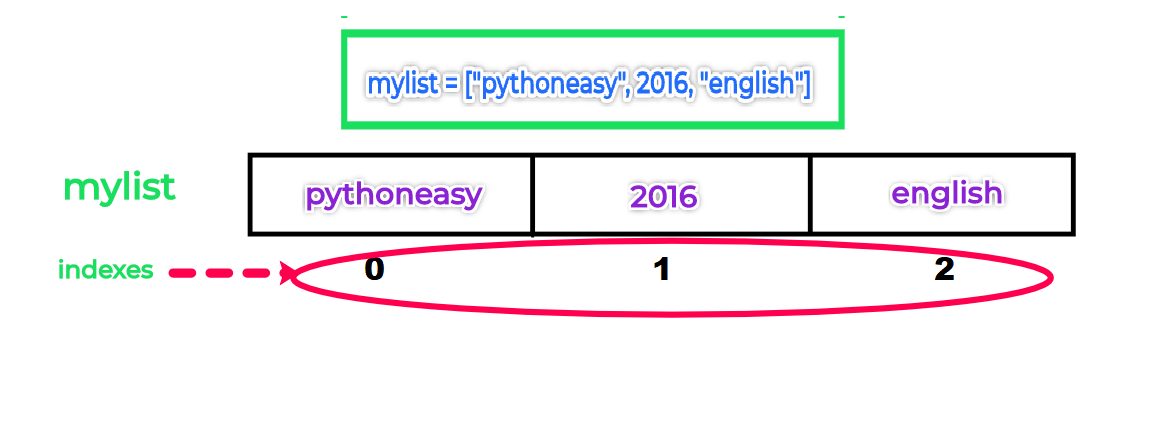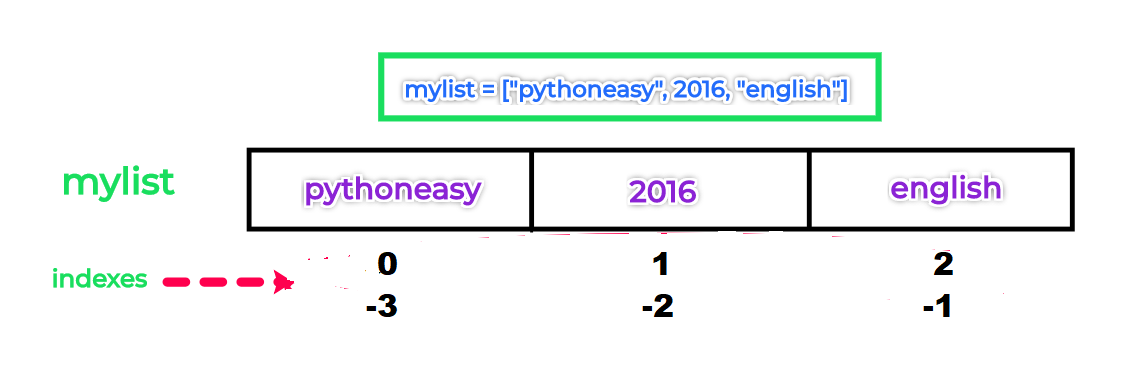# Python Lists

## What is Python list ?

Python lists are positionally (by index) ordered collections of arbitrarily typed objects (Items can be any type)
Python lists have no fixed size.
Lists are mutable type that means they can be changed after assigned.

## How to create List ?

Python lists can be created using square brackets [] or list() built-in function
Example 1 :

``````mylist = ["pythoneasy", 2016, "english"]
print(type(mylist))
``````
<class 'list'>
We can pass an iterable (collection data type like tuple or dictionary) to list() function to create a list
Example 2 :
``````tuple1 = ("pythoneasy", 2016, "english")
mylist = list(tuple1)
print(mylist)``````
['pythoneasy', 2016, 'english']

### Accessing list values or objects

As list is a sequence data type, the items or objects are stored in the memory in the sequence with index starting from 0 (as shown in the below image)so each element is referred to a index.
In the above example "pythoneasy" is linked to positional index 0 and 2016 to 1 and "english" to 2
We can access these with reference mylist, mylist and mylist. This process is also known as indexing

``````mylist = ["pythoneasy", 2016, "english"]
print(mylist) # prints "pythoneasy"
print(mylist) # prints 2016
print(mylist) # prints "english"
``````
pythoneasy
2016
english

### Changing list values

Using the indexes we can also change the values/objects inside the list

``````mylist = ["pythoneasy", 2016, "english"]
mylist = 2018            # Changes the second object
print(mylist)
mylist = "python.org"    # Changes the first object
mylist = "spanish"       # Changes the third object
print(mylist)
``````
['pythoneasy', 2018, 'english']
['python.org', 2018, 'spanish']

While using index like above you need to be careful about the number of index.
As we have 3 objects/items here the maximum index will be 2 .i.e. length of the list - 1
Trying to index a value more than this will cause an IndexError
Example:

``````mylist = ["pythoneasy", 2016, "english"]
mylist = 2018 # index more than maximum index
``````
Traceback (most recent call last): File "D:/pythoneasy/list_example.py", line 2, in <module> mylist = 2018 IndexError: list assignment index out of range

### Length of a list

The built-in function len() helps to determine the length of a list

``````mylist = ["pythoneasy", 2016, "english"]
print(len(mylist))
``````

3
The last index of index can be calculated as len(mylist) -1

``````mylist = ["pythoneasy", 2016, "english"]
print(mylist[len(mylist) - 1])
``````

english
so `len(mylist) - 1]` provides 2 and `mylist` results in "english"

### Negative index

Python also supports negative index.
When we create a list, the negative index will be created from right to left starting from -1 (as shown in the below image)``````mylist = ["pythoneasy", 2016, "english"]
``````

In the above example mylist[-1] provides the last value always which is "english"
And the value of mylist[-3] is same as mylist and mylist[-2] as mylist

``````print(mylist[-1])
``````
english

### Slicing

We can also extract a range of a items from a list using indexes know as slicing.
The syntax is

``````variable_name[I:J] # Gives everything in variable_name from I to J, but inot including J
``````

Example:

``````mylist = ["pythoneasy", 2016, "english", 2017, 2018]
print(mylist[1:4])
``````

[2016, 'english', 2017]
So it extracts the a list from offset/index 1 to 3 (Not including 4)
Slicing also can be done using built in function slice()
Example:

``````mylist = ["pythoneasy", 2016, "english", 2017, 2018]
print(mylist[slice(1, 4)])
``````

[2016, 'english', 2017]
`slice(1, 4)` is same as `1:4` , when provided to list, it gives a list of two element on index 1 to 3

## List Comprehension

List comprehension provide a concise way to create lists.
Using list comprehension expression we can create a new list using another list.
Syntax :

``````[ output_expression for(set of values to iterate) if(conditional filtering) ]
``````

ExamplesLet us create a list containing only even numbers from another iterable using for loop.

Example 1:

``````tuple_1 = (1, 2, 3, 4, 5, 6, 7, 8, 9)
list_1 = list()
for element in tuple_1:
if element % 2 == 0:
list_1.append(element)
print(list_1)
``````

[2, 4, 6, 8]
In the above program, list_1 is created from tuple_1 using for loop.
The above program can be done using list comprehension expression as follows

Example 2:

``````tuple_1 = (1, 2, 3, 4, 5, 6, 7, 8, 9)
list_1 = [element for element in tuple_1 if element % 2 == 0] # in a single line
print(list_1)
``````

In the above program we converted the whole for loop block to a single line using list comprehension expression to create list_1

For loop works as

``````for (set of values to iterate):
if (conditional filtering):
output_expression
``````

The above for loop can be written using List comprehension as

``````[ output_expression for(set of values to iterate) if(conditional filtering) ]
``````

Note: List comprehension mechanism is faster than for loops

## Python List methods

Python has a set of built-in methods that you can use on lists
This is equivalent to a[len(a):] = [x]
Example:
``````>>> list_1 = [1, 2, 3, 'hello']
>>> list_1.append('user')
>>> list_1
[1, 2, 3, 'hello', 'user']```
```
In the above code 'user' is added to list_1
This is equivalent to a[len(a):] = iterable
Example:
``````>>> list_1 = [1, 2, 3, 'hello']
>>> list_1.extend(['user', 'name'])
>>> list_1
[1, 2, 3, 'hello', 'user', 'name']```
```
In the above code ['user', 'name'] is added to list_1
Example:
``````>>> list_1 = [71, 56, 91, 'hello']
>>> list_1.insert(2, 'user')
>>> list_1
[71, 56, 'user', 91, 'hello']```
```
In the above code 'user' is inserted to index '2'
Example:
``````>>> list_1 = [71, 56, 91, 'hello']
>>> list_1.remove(91)
>>> list_1
[71, 56, 'hello']
>>> list_1.remove(34)
Traceback (most recent call last):
File "<stdin>", line 1, in <module>
ValueError: list.remove(x): x not in list```
```
list_1.remove(91) removes 91 from the list.
But remove() raises ValueError if element is not present in the list
So list_1.remove(34) raises ValueError
Example:
``````>>> list_1 = [71, 56, 91, 'hello']
>>> list_1.pop(1)
56
>>> list_1
[71, 91, 'hello']``````
list_1.pop(1) pops out element 56
This is same as
``del list_1``

Example:
``````>>> list_1 = [71, 56, 91, 'hello']
>>> list_1.clear()
>>> list_1
[]``````
Raises a ValueError if there is no such item.
Example:
``````>>> list_1 = [71, 56, 91, 'hello']
>>> list_1.index(56)
1
>>> list_1.index(15)
Traceback (most recent call last):
File "<stdin>", line 1, in <module>
ValueError: 15 is not in list
>>>``````
56 is at index 1, so list_1.index(56) prints 1
list_1.index(15) raises ValueError as there is no element with value 15 in the list
Example:
``````>>> list_1 = [71, 56, 91, 71, 2, 82, 71, 'hello']
>>> list_1.count(71)
3``````
71 is present 3 times in list_1
Example:
Example:
``````>>> list_1 = [71, 56, 91, 'hello']
>>> list_1.reverse()
>>> list_1
['hello', 91, 56, 71]``````
Example:
``````>>> list_1 = [71, 56, 91, 'hello']
>>> list_2 = list_1.copy()
>>> list_2
[71, 56, 91, 'hello']``````